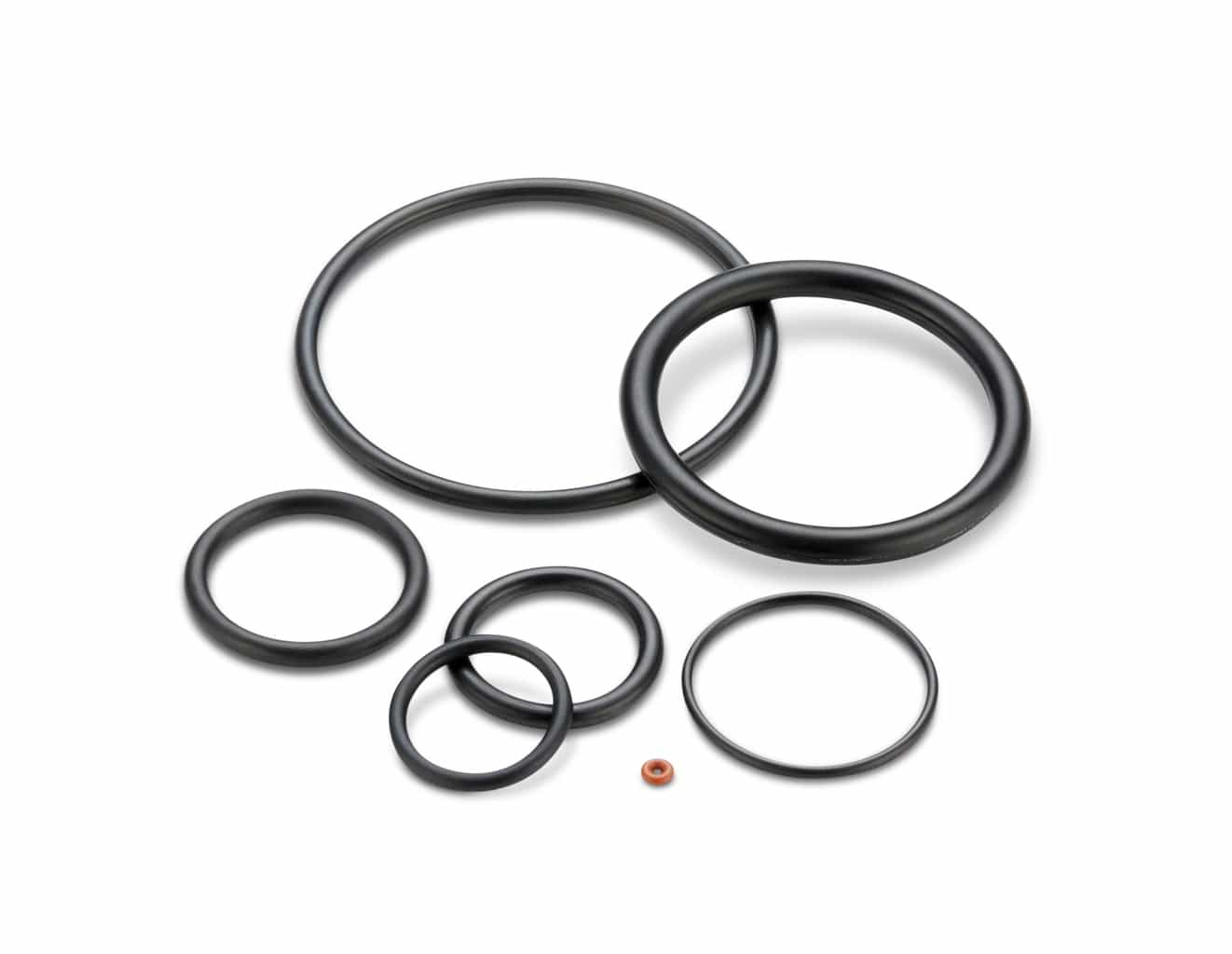# Quad-Ring® Face Seal Application Example

## Application description: Cover for a Static Pressure Vessel, U. S. Customary Units (inches)

• Inside pressure of 50 psi
• Bore diameter 0.500″ ±0.005
• Desired Maximum groove OD of 0.750″ -0.005/+0.000
• 0.103″ cross-section Quad-Ring® Brand seal
• Cover is flat

1. Determine the groove depth:
Since the cover is flat, the groove depth is simply the gland depth. For this static application, the recommended gland depth from the table is 0.089.
Groove Depth = Gland Depth = 0.089 -0.002/+0.000
For the purpose of this example, a tolerance on this dimension of -0.002/+0.000 is assumed.

2. Calculate the groove inside diameter.
From the table, the groove width for a 0.103 cross-section seal is 0.115 -0.000/+0.005.
Groove I.D. = Minimum Groove O.D. – (2 x Groove Width)
= 0.745 – (2 X 0.115)
= 0.515 -0.005/+0.000
For the purpose of this example, a tolerance on this dimension of -0.000/+0.005 is assumed.

3. Calculate the Minimum Gland Cross-sectional Area:
Minimum Gland Volume = ((Min Groove O.D. – Max Groove I.D.) / 2) x Min Gland Depth
= ((0.745 – 0.515 )/2) X 0.087
= 0.010 in2

4. Calculate the Maximum Quad-Ring® Brand Seal Cross-sectional Area:
Seal Volume = (Max Quad-Ring® Brand Cross-section)2 X 0.8215
= (0.106)2 X 0.8215
= 0.00923 in2

5. Compare the Minimum Gland Volume to the Maximum Quad-Ring® Brand Seal Volume
In this application the maximum seal volume is less than the minimum gland volume, so the seal should function satisfactorily.

6. Calculate the Minimum and Maximum Seal Squeeze
a.
Max Seal Squeeze = 1 – (Min Gland Depth / Max Seal Cross-section
= 1 – (0.087 / 0.106)
= 0.179 = 17.9%
b. Min Seal Squeeze = 1 – (Max Gland Depth / Min Seal Cross-section)
= 1 – (0.089/.100)
= 0.11 = 11%
Therefore, sufficient squeeze should exist to seal this application.

7. Select the Seal Size
Refer to the Selection Guide and turn to the section which lists the seals having a 0.103 cross-section. Since this is an internal pressure application, the seal OD should always be seated against the groove OD, which has a maximum size of 0.750. Since the Selection Guide Table provides seal ID information, determine the minimum required ID by subtracting the minimum seal cross-section:
Min ID= 0.750 – 2 x Min seal Cross-section = 0.750 X (2 X 0.100) = 0.550
A 4114 seal would always have a minimum ID greater than 0.550.